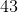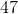# Common Core: 1st Grade Math : Understanding Place Value

## Example Questions

### Example Question #71 : Understanding Place Value

What digit is in the ones place?Explanation:

The ones place is always the first number on the right.

### Example Question #72 : Understanding Place Value

What digit is in the ones place?Explanation:

The ones place is always the first number on the right.

### Example Question #73 : Understanding Place Value

What digit is in the ones place?Explanation:

The ones place is always the first number on the right.

### Example Question #74 : Understanding Place Value

What digit is in the ones place?Explanation:

The ones place is always the first number on the right.

### Example Question #73 : Understanding Place Value

What digit is in the ones place?Explanation:

The ones place is always the first number on the right.

### Example Question #74 : Understanding Place Value

What digit is in the ones place?Explanation:

The ones place is always the first number on the right.

### Example Question #75 : Understanding Place Value

What digit is in the ones place?Explanation:

The ones place is always the first number on the right.

### Example Question #76 : Understanding Place Value

What digit is in the ones place?Explanation:

The ones place is always the first number on the right.

### Example Question #77 : Understanding Place Value

What digit is in the ones place?Explanation:

The ones place is always the first number on the right.

### Example Question #81 : Understanding Place Value

What digit is in the ones place?